Courses

# Worksheet - Shapes and Angles Notes | EduRev

## Class 5 : Worksheet - Shapes and Angles Notes | EduRev

The document Worksheet - Shapes and Angles Notes | EduRev is a part of the Class 5 Course Mathematics for Class 5: NCERT.
All you need of Class 5 at this link: Class 5

(a)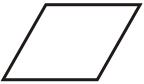Ans.
Rhombus

(b)Ans.
Square

(c)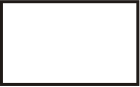Ans.
Rectangle

(d)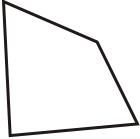Ans.

(e)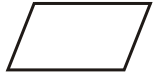Ans.
Parallelogram

(f)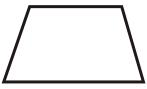Ans.
Trapezoid

Q.2. Fill in the blanks:
(a) 1/3 of a right angle is ____
Ans.
30 degree

(b) The number of right angles in a square is ____
Ans.
4

(c) The clock time at which the hands of a clock forms an acute angle is ____
Ans.
2’O Clock

(d) Two times of a right angle is ____
Ans.
180 degree or straight line.

(e) ____ is used to measure an angle.
Ans.
Protractor

(f) Draw a clock showing the time 3’O clock and write the angle indicated on the clock face.
Ans.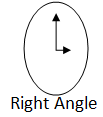(g) Write four English alphabets where you can see a right angle.
Ans.
E, F, H, L

(h) Write Sanu’s name by straight lines. Mark all the angles as acute, obtuse or right angle.
Ans.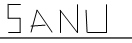(i) The number of right angles in S is 4.

(ii) The number of acute angle in A is 3 and obtuse angle is 2.

(iii) The number of acute angle in N is 2.

(iv) The number of right angles in U is 2.

(v) Total number of right angles = 6

(vi) Total number of acute angles = 5.

(vii) Total number of obtuse angle =2

(i) Draw an acute angle and name it.
Ans.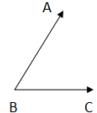(j) Draw the positions of the hands of a clock at the given time and write the name of the angle thus formed Time: 2’O Clock
Ans.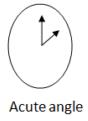Q.3.
(a) A train leaves the station at 9.30 am and reaches its destination at 5.45 pm. Find the duration of the journey.
Ans.
8 hours 15 minutes

(b) Convert into seconds: 7 minutes 55 seconds
Ans.
475 seconds

(c) Fill in the blanks: Freezing Point of water = ________ °C = ______ °F
Ans.
0, 32

(d) What time will it be: 5 hours 15 minutes after 2250 hours?
Ans.
04:50 am

(e) The maximum temperature of Noida on last Monday was 37.2 degree C while the minimum temperature was 15.4 degree C. What was the difference in temperature?
Ans.
21.8 degree C

(f) Geeta joined dance classes on 22 April for 25 days. Which date is the last class for her?
Ans.
17 May

(g) State the kind of weather when the temperature is : 4 degree Celsius
Ans.
Cold

(h) Complete the following: If starting date is 5 April and the duration is 35 days, the ending date will be _________.
Ans.
10 May

(i) Fill in the blanks: 4 minutes 15 seconds = ________ seconds
Ans.
255

(j) What time will it be: 4 hours 25 minutes before 2:05 am?
Ans.
9:40 pm

Offer running on EduRev: Apply code STAYHOME200 to get INR 200 off on our premium plan EduRev Infinity!

## Mathematics for Class 5: NCERT

40 videos|43 docs|39 tests

,

,

,

,

,

,

,

,

,

,

,

,

,

,

,

,

,

,

,

,

,

;